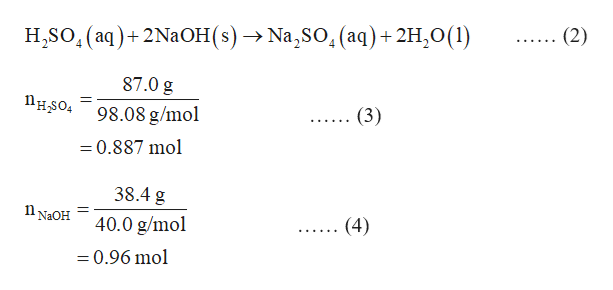Aqueous sulfuric acidH2SO4will react with solid sodium hydroxideNaOHto produce aqueous sodium sulfateNa2SO4and liquid waterH2O.Suppose 87. g of sulfuric acid is mixed with 38.4 g of sodium hydroxide. Calculate the maximum mass of water that could be produced by the chemical reaction. Be sure your answer has the correct number of significant digits.

Question

Aqueous sulfuric acid

H2SO4

will react with solid sodium hydroxide

NaOH

to produce aqueous sodium sulfate

Na2SO4

and liquid water

H2O

.Suppose 87. g of sulfuric acid is mixed with 38.4 g of sodium hydroxide. Calculate the maximum mass of water that could be produced by the chemical reaction. Be sure your answer has the correct number of significant digits.

Step 1

Limiting reagent is defined as a reactant which is present in limited quantity in the reaction. The reaction ceases after the consumption of the limiting reagent.

The theoretical yield of the product formed in the reaction is calculated by considering the number of moles of the limiting reagent.

The number of moles of a species is calculated by the expression (1) in which n is the number of moles, m is the mass and M is the molar mass of the species.

Step 2

The given reaction is represented in the equation (2).

The number of moles of sulfuric acid and sodium hydroxide in the reaction are calculated in the equations (3) and (4) respectively.help_outlineImage TranscriptioncloseH.SO, (aq)+ 2NaOH (s) —> Na,SO, (аq) + 2H,0(1) (2) вв . 87.0 g Пнsо 98.08 g/mo (3) =0.887 mol 38.4 g n NaOH 40.0 g/mol (4) =0.96 mol fullscreen
Step 3

In the reaction (2), one mole of sulfuric acid reacts with 2 moles of sodium hydroxide.

This means 0.48 moles of sulfuric acid are required for 0.96 mol of sodium hydroxide. But the number of ...

Want to see the full answer?

See Solution

Want to see this answer and more?

Our solutions are written by experts, many with advanced degrees, and available 24/7

See Solution
Tagged in

General Chemistry